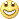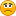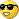# Daily Breakout EA - page 924

going live with hilo4

after backtesting, going live.52

which EA r u using ?

I did backtests too and all 4 EA's crashed the accountcould u please post your settings ?

rgds63

hova:
movetoBEP means that EA is moving SL to Entry when the pos. is in a certain amount of profit. if i were u, i would run this EA on a demo acc for at least a month

Ok... but were do i decide how many pips in profit before moving SL to BE? The parameter below movetoBEP is named: 'LockProfit' and 'TrailingStop' non of these sounds to be the logic parameter to set the movetoBEP.

And then we stil have 'addpoint' and 'Lockprofit'.

But is quite funny... only hova seems to have any clue of what the parameters means... yet there are way more then hova in this thread....

Regards / JoLi52

jolimoney:
Ok... but were do i decide how many pips in profit before moving SL to BE? The parameter below movetoBEP is named: 'LockProfit' and 'TrailingStop' non of these sounds to be the logic parameter to set the movetoBEP.

And then we stil have 'addpoint' and 'Lockprofit'.

But is quite funny... only hova seems to have any clue of what the parameters means... yet there are way more then hova in this thread....

Regards / JoLi

im not a coder, so i cant review the whole code, some more comments would make it easy to understand these things

*looking 4 the coder*63

hova:
im not a coder, so i cant review the whole code, some more comments would make it easy to understand these things *looking 4 the coder*Agree with hova. If only someone with the knowledge of these parameters can explain the parameters for us we could all help to find the best settings for it and that would be profitable for us all.

Regards / JoLi52

Rangepoint:

primajaya:
"Actually You may use this EA from TF M15 to Daily TF, but when you use this EA in lower Period, you may find that High and Low of previos bar is less than stop level, so the EA cannot send stop order. Because of that, if the distance of high and low is less than the stoplevel, we will make adjustment for the distance by using range point"63

hova:
Rangepoint:

Originally Posted by primajaya

"Actually You may use this EA from TF M15 to Daily TF, but when you use this EA in lower Period, you may find that High and Low of previos bar is less than stop level, so the EA cannot send stop order.

Because of that, if the distance of high and low is less than the stoplevel, we will make adjustment for the distance by using range point"

Yes... it seems logic. With Rangepoint we add X pips to each side for a breakout to happen.

But then again we have 'addpoint' and 'lockprofit' which we stil not have any clue of what they do. And the 'MovetoBEP' is stil a mystery... when do the EA move to breakeven.

ergards / JoLi52

Addpoint :

I think, if yesterdays-High and yesterdays-Low are too close to each other, stop loss of high and low would be above the other order ( stop loss of short above limit buy) and this is where Addpoint comes in.

In the case that stop loss of the short order, Addpoint would decal the givin points.

So if stop loss is 1.5000 and limit buy would be 1.4995 (assuming that yesterdays high is 1.4985) Addpoint would place stop order @ 1.4975 (standard setting Addpoint = 10)

EA Code is following:

if(High-Ask < stoplevel) buyPrice = Ask + RangePoint*point();

else buyPrice = High + AddPoint*point();

if(Bid-Low < stoplevel) sellPrice = Bid - RangePoint*point();

else sellPrice = Low - AddPoint*point();

Correct me if im wrong, but i think thats it.

Conclusion about BEP + LockProfit following these days...24

hilo_4

leanne:
after backtesting, going live.!

backtesting looks good, however today look like a stop out.

version 4, set file is the one someone posted on this thread, with sl 80 and trailing 15. (i have done backtesting with sl 40, not very good. also the original setting with tp20 and sl20 is no good). i copy it for you here:

TP=200

TP,F=1

TP,1=20

TP,2=0

TP,3=0

TP_Increment=35

TP_Increment,F=1

TP_Increment,1=20

TP_Increment,2=0

TP_Increment,3=0

SL=80

SL,F=1

SL,1=80

SL,2=0

SL,3=0

RangePoint=10

RangePoint,F=0

RangePoint,1=10

RangePoint,2=0

RangePoint,3=0

AddPoint=3

AddPoint,F=1

AddPoint,1=10

AddPoint,2=0

AddPoint,3=0

LotSize=0.50000000

LotSize,F=1

LotSize,1=1.00000000

LotSize,2=0.00000000

LotSize,3=0.00000000

LotDevider=5

LotDevider,F=1

LotDevider,1=5

LotDevider,2=0

LotDevider,3=0

MagicNumber=123456789

MagicNumber,F=0

MagicNumber,1=123456789

MagicNumber,2=0

MagicNumber,3=0

DelOpposite=0

MoveToBEP=1

LockProfit=1

LockProfit,F=0

LockProfit,1=1

LockProfit,2=0

LockProfit,3=0

TrailingStop=15

TrailingStop,F=1

TrailingStop,1=40

TrailingStop,2=0

TrailingStop,3=0

hope this will help24

for hova

hova:
which EA r u using ?

I did backtests too and all 4 EA's crashed the accountcould u please post your settings ?

rgds

sorry hova, my previous post was meant to reply to your post. however the quote went missing.